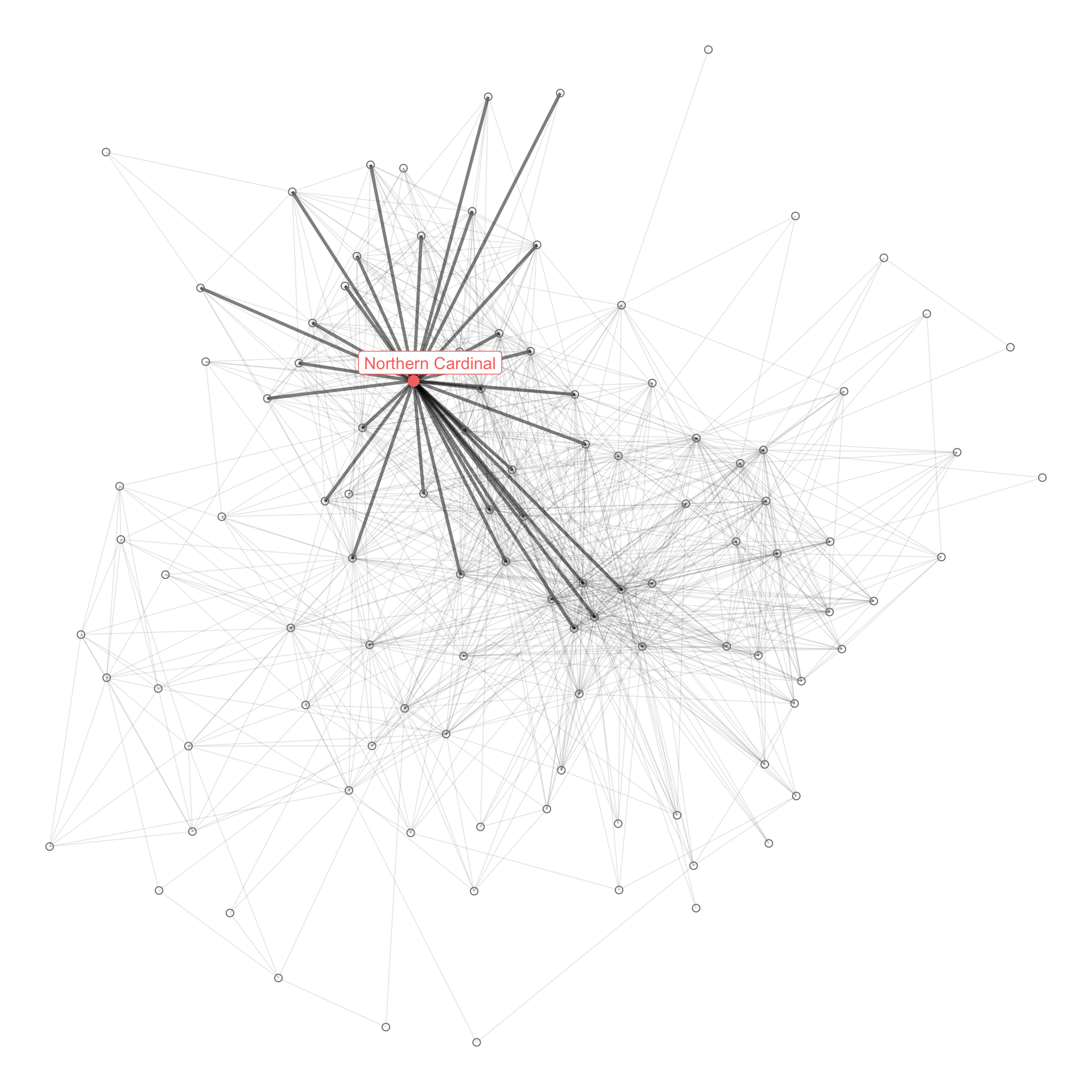# eBirding in Allegheny County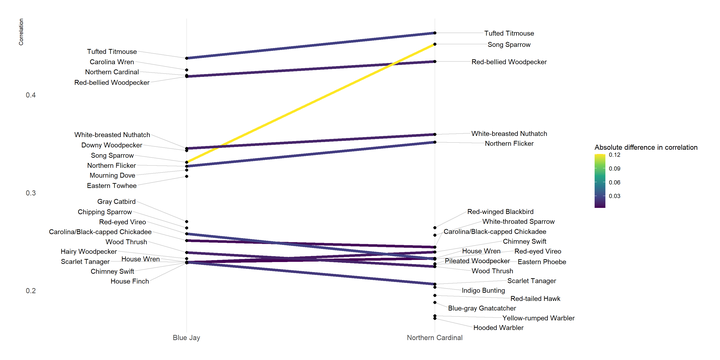In this post I will do some exploratory analysis on eBird data. I’ve picked up birdwatching as a hobby during quarantine, and eBird has a ton of cool data on bird sightings.

## Setup

``````library(tidyverse)
library(lubridate)
library(vroom)
library(janitor)
library(rebird)
library(hrbrthemes)
library(ggrepel)
library(gganimate)
library(widyr)
library(tidygraph)
library(ggraph)
library(tidytext)

options(scipen = 999, digits = 2)

theme_set(theme_ipsum())

set.seed(1234)``````

## Load and filter data

I downloaded data for bird sightings in Allegheny County from the eBird data portal. This code loads the data in R and prepares it for analysis.

``````df <- vroom("data/ebd_US-PA-003_201001_202003_relFeb-2020.zip", delim = "\t") %>%
clean_names() %>%
mutate_at(vars(observer_id, locality, observation_date, time_observations_started, protocol_type), str_replace_na, "NA") %>%
mutate(observation_date = ymd(observation_date),
observation_count_old = observation_count,
observation_count = as.numeric(str_replace(observation_count_old, "X", as.character(NA))),
observation_event_id = str_c(observer_id, locality, observation_date, time_observations_started)) %>%
filter(all_species_reported == 1)``````

I will focus on the two major “types” of observation protocols. The others are related to specific birding events, and might not be representative of the overall data.

``````df_top_protocols <- df %>%
count(protocol_type, sort = TRUE) %>%
slice(1:2)

df_top_protocols``````
``````## # A tibble: 2 x 2
##   protocol_type      n
##   <chr>          <int>
## 1 Traveling     318714
## 2 Stationary    178252``````
``````df <- df %>%
semi_join(df_top_protocols)``````

This shows that Allegheny County birders began submitting data regularly in 2016. I will focus my analysis on recent observations.

``````df %>%
count(observation_date) %>%
mutate(recent_observation = year(observation_date) >= 2016,
observation_date = ymd(observation_date)) %>%
ggplot(aes(observation_date, n, color = recent_observation)) +
geom_line() +
scale_y_comma() +
scale_color_discrete("Recent Observation") +
labs(y = "Number of observations",
x = "Observation date")``````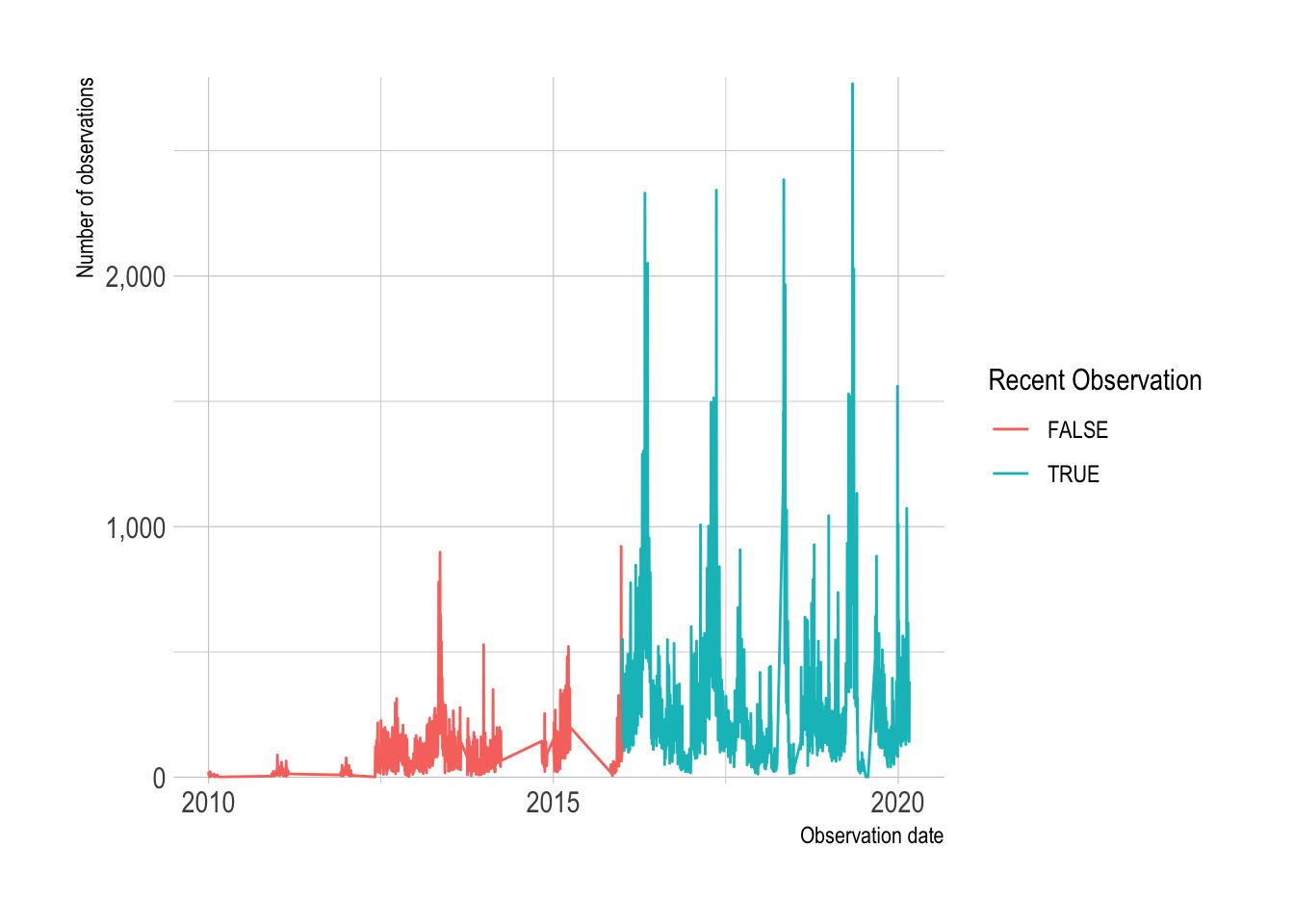``````df <- df %>%
filter(year(observation_date) >= 2016)``````

There is wide variation in the number of times a species was sighted in a given observation.

``````df %>%
ggplot(aes(observation_count, group = common_name)) +
geom_density() +
scale_x_log10() +
labs(x = "Species count (log10)")``````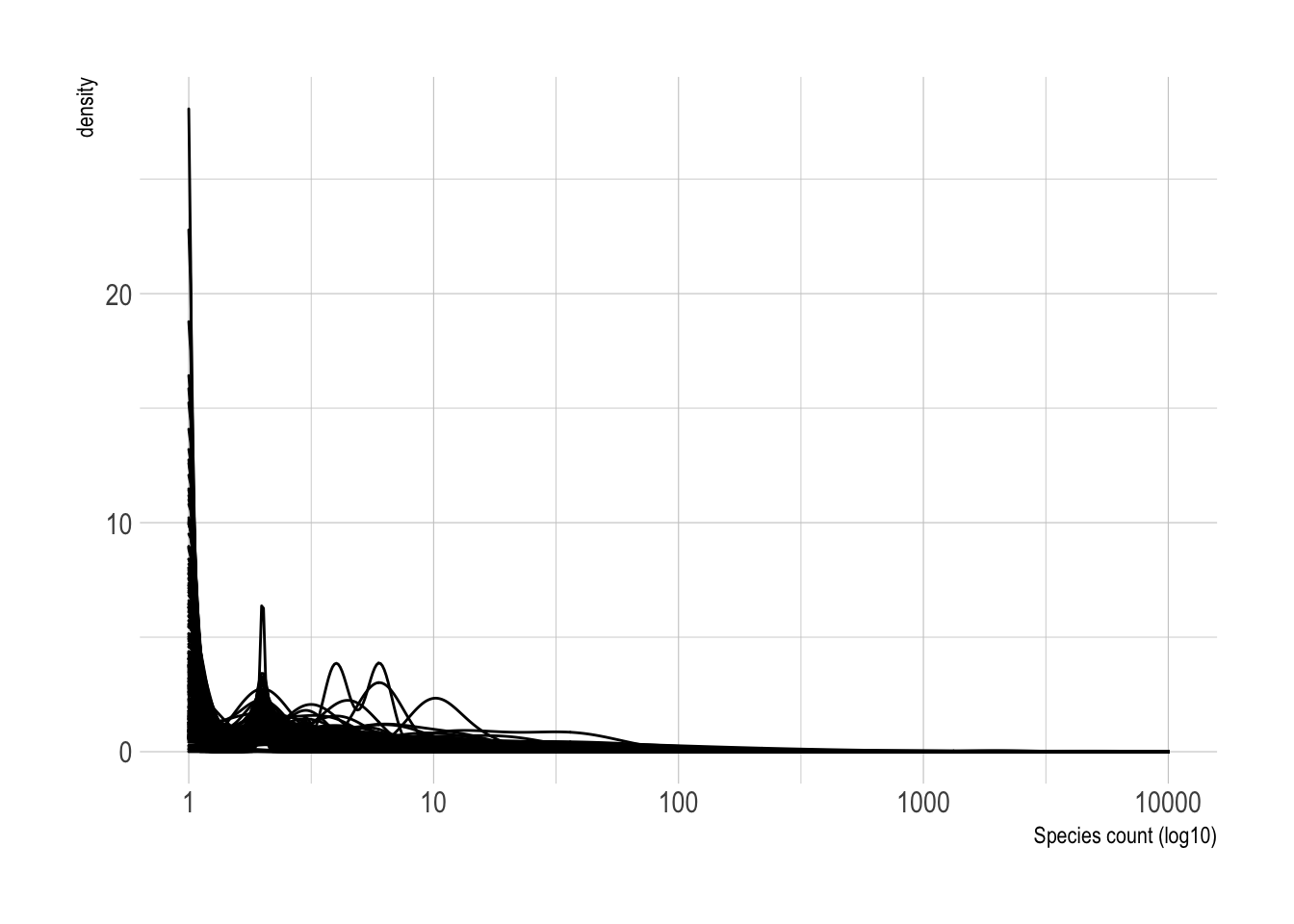This calculates the 99th percentile of bird count per observation, and highlights the birds that are seen in large flocks.

``````df %>%
group_by(common_name) %>%
summarize(observation_count_99 = quantile(observation_count, probs = c(.99), na.rm = TRUE)) %>%
arrange(desc(observation_count_99))``````
``````## # A tibble: 292 x 2
##    common_name       observation_count_99
##    <chr>                            <dbl>
##  1 Ring-billed Gull                 4437.
##  2 crow sp.                         2000
##  3 gull sp.                          696.
##  4 Tundra Swan                       231.
##  5 European Starling                 200
##  6 Herring Gull                      200
##  7 Canada Goose                      148
##  8 Common Nighthawk                  142.
##  9 blackbird sp.                     103.
## 10 Mallard                           101.
## # … with 282 more rows``````

This shows that the high count of Ring-billed Gulls is explained by groups of birders seeing flocks of thousands of the species. This also highlights that the same bird sighting can be counted twice because of simultaneous observation.

``````df %>%
filter(common_name == "Ring-billed Gull") %>%
arrange(desc(observation_count)) %>%
slice(1:10) %>%
select(observer_id, group_identifier, common_name, observation_date, duration_minutes, observation_count, locality)``````
``````## # A tibble: 10 x 7
##    observer_id group_identifier common_name observation_date duration_minutes
##    <chr>       <chr>            <chr>       <date>                      <dbl>
##  1 obsr160352  G2943178         Ring-bille… 2018-02-13                     38
##  2 obsr40545   G2943178         Ring-bille… 2018-02-13                     38
##  3 obsr40545   G2930894         Ring-bille… 2018-02-09                     50
##  4 obsr160352  G2930894         Ring-bille… 2018-02-09                     75
##  5 obsr160352  <NA>             Ring-bille… 2016-01-29                     25
##  6 obsr160352  G1578214         Ring-bille… 2016-01-30                     71
##  7 obsr101818  G1578214         Ring-bille… 2016-01-30                     71
##  8 obsr40545   G2940041         Ring-bille… 2018-02-12                     55
##  9 obsr160352  <NA>             Ring-bille… 2018-02-10                     55
## 10 obsr620338  G2940041         Ring-bille… 2018-02-12                     55
## # … with 2 more variables: observation_count <dbl>, locality <chr>``````

## Species counts

This does a basic count of the major species, not accounting for simultaneous observation:

``````df_counts <- df %>%
group_by(common_name) %>%
summarize(species_count = sum(observation_count, na.rm = TRUE)) %>%
arrange(desc(species_count))

df_counts``````
``````## # A tibble: 292 x 2
##    common_name       species_count
##    <chr>                     <dbl>
##  1 Ring-billed Gull         216490
##  2 European Starling        151465
##  3 American Crow            143783
##  4 Canada Goose             124268
##  5 American Robin           114062
##  6 Mallard                   78072
##  7 Northern Cardinal         68276
##  8 Mourning Dove             56251
##  9 Common Grackle            51649
## 10 Blue Jay                  49700
## # … with 282 more rows``````
``````df_counts %>%
mutate(common_name = fct_reorder(common_name, species_count)) %>%
slice(1:20) %>%
ggplot(aes(species_count, common_name)) +
geom_col() +
scale_x_comma() +
labs(x = "Observations",
y = NULL)``````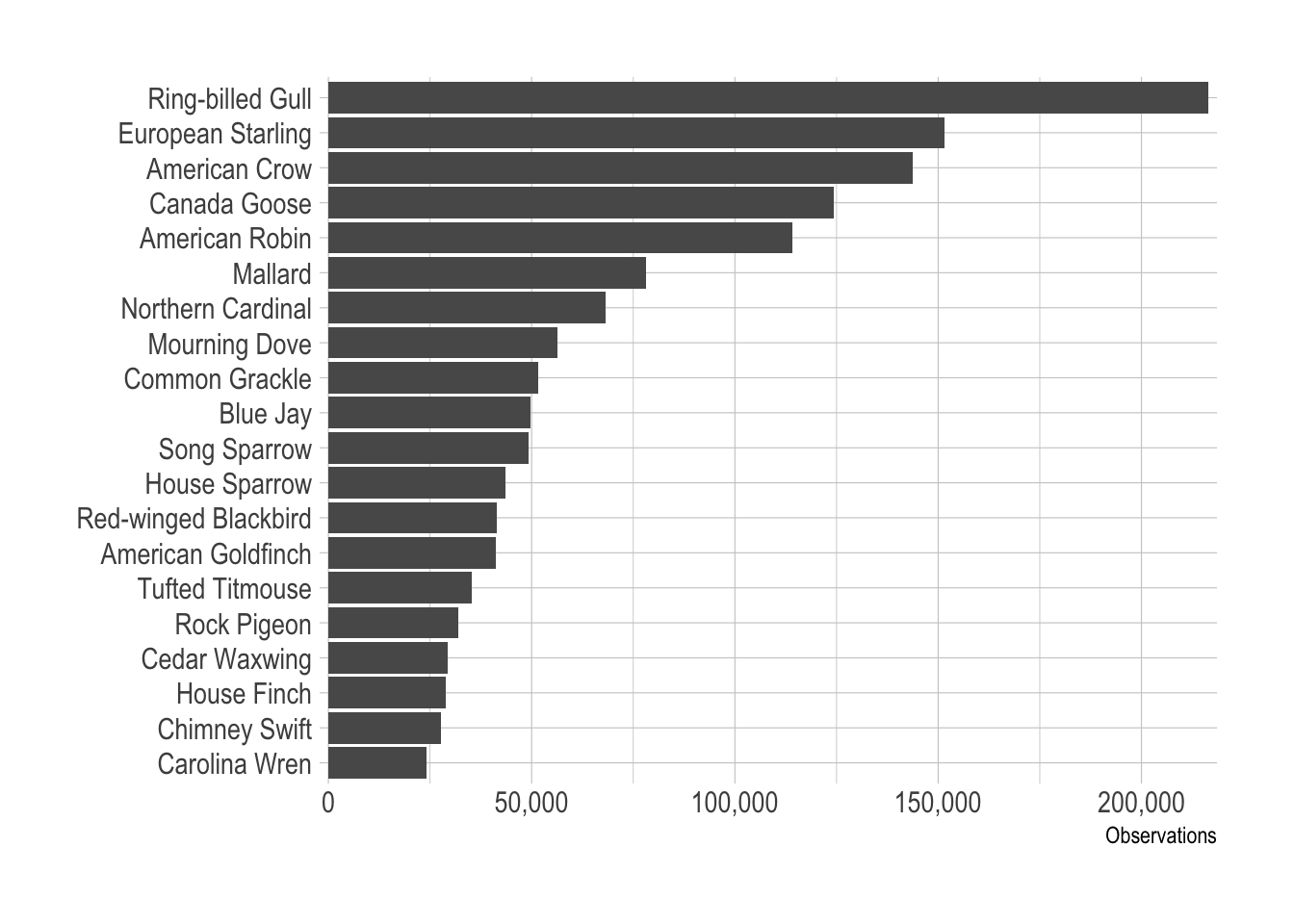## Species correlations

I was interested in which birds are correlated most with two common and popular birds, the Cardinal and the Blue Jay. This calculates the pairwise correlation and plots the top 10 birds correlated with the two target species:

``species_list <- c("Northern Cardinal", "Blue Jay")``
``````df_pair_corr <- df %>%
pairwise_cor(common_name, observation_event_id, diag = FALSE, upper = FALSE)``````
``````df_pair_corr %>%
filter(item1 %in% species_list) %>%
drop_na(correlation) %>%
arrange(item1, desc(correlation)) %>%
group_by(item1) %>%
slice(1:10) %>%
ungroup() %>%
mutate(item2 = reorder_within(x = item2, by = correlation, within = item1)) %>%
ggplot(aes(correlation, item2, fill = item1)) +
geom_col(alpha = .9) +
facet_wrap(~item1, scales = "free_y") +
scale_y_reordered() +
scale_fill_manual(values = c("blue", "red")) +
guides(fill = FALSE) +
labs(x = "Correlation",
y = NULL)``````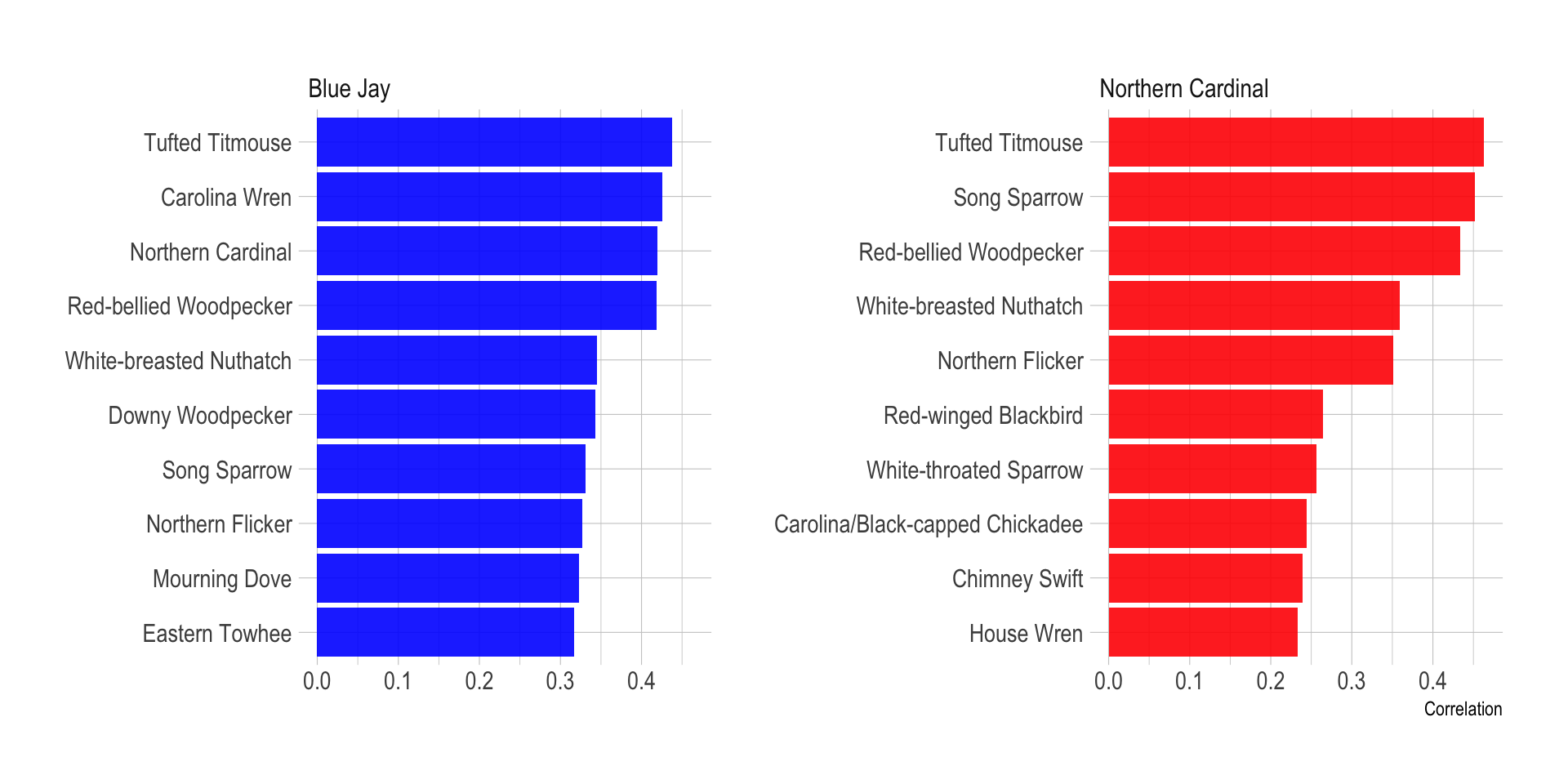Cardinals and Blue Jays share many birds in common, but there are some distinctions, and the level of correlation can vary. I use a slopegraph to show the difference in how much a bird is correlated with Blue Jays vs. Cardinals.

This grabs the data for the two target species and gets the top 20 correlated birds for each species:

``````df_slopegraph <- df_pair_corr %>%
filter(item1 %in% species_list) %>%
drop_na(correlation) %>%
arrange(item1, desc(correlation)) %>%
group_by(item1) %>%
slice(1:20) %>%
ungroup()``````

This calculates the difference in correlation for each bird:

``````df_corr_diff <- df_slopegraph %>%
pivot_wider(names_from = item1, values_from = correlation, names_prefix = "corr_") %>%
clean_names() %>%
mutate(corr_diff = abs(corr_blue_jay - corr_northern_cardinal)) %>%
select(item2, corr_diff)

df_corr_diff``````
``````## # A tibble: 29 x 2
##    item2                   corr_diff
##    <chr>                       <dbl>
##  1 Tufted Titmouse            0.0258
##  2 Carolina Wren             NA
##  3 Northern Cardinal         NA
##  4 Red-bellied Woodpecker     0.0151
##  5 White-breasted Nuthatch    0.0143
##  6 Downy Woodpecker          NA
##  7 Song Sparrow               0.121
##  8 Northern Flicker           0.0245
##  9 Mourning Dove             NA
## 10 Eastern Towhee            NA
## # … with 19 more rows``````
``````df_slopegraph %>%
left_join(df_corr_diff) %>%
ggplot(aes(item1, correlation)) +
geom_line(aes(group = item2, color = corr_diff), size = 2) +
geom_point(size = 2) +
geom_text_repel(data = filter(df_slopegraph, item1 == species_list),
aes(y = correlation, label = item2), direction = "both", nudge_x = -.3, segment.alpha = .2) +
geom_text_repel(data = filter(df_slopegraph, item1 == species_list),
aes(y = correlation, label = item2), direction = "both", nudge_x = .3, segment.alpha = .2) +
scale_color_viridis_c("Absolute difference in correlation") +
labs(x = NULL,
y = "Correlation") +
theme(panel.grid.minor.y = element_blank(),
panel.grid.major.y = element_blank(),
axis.title.x = element_blank())``````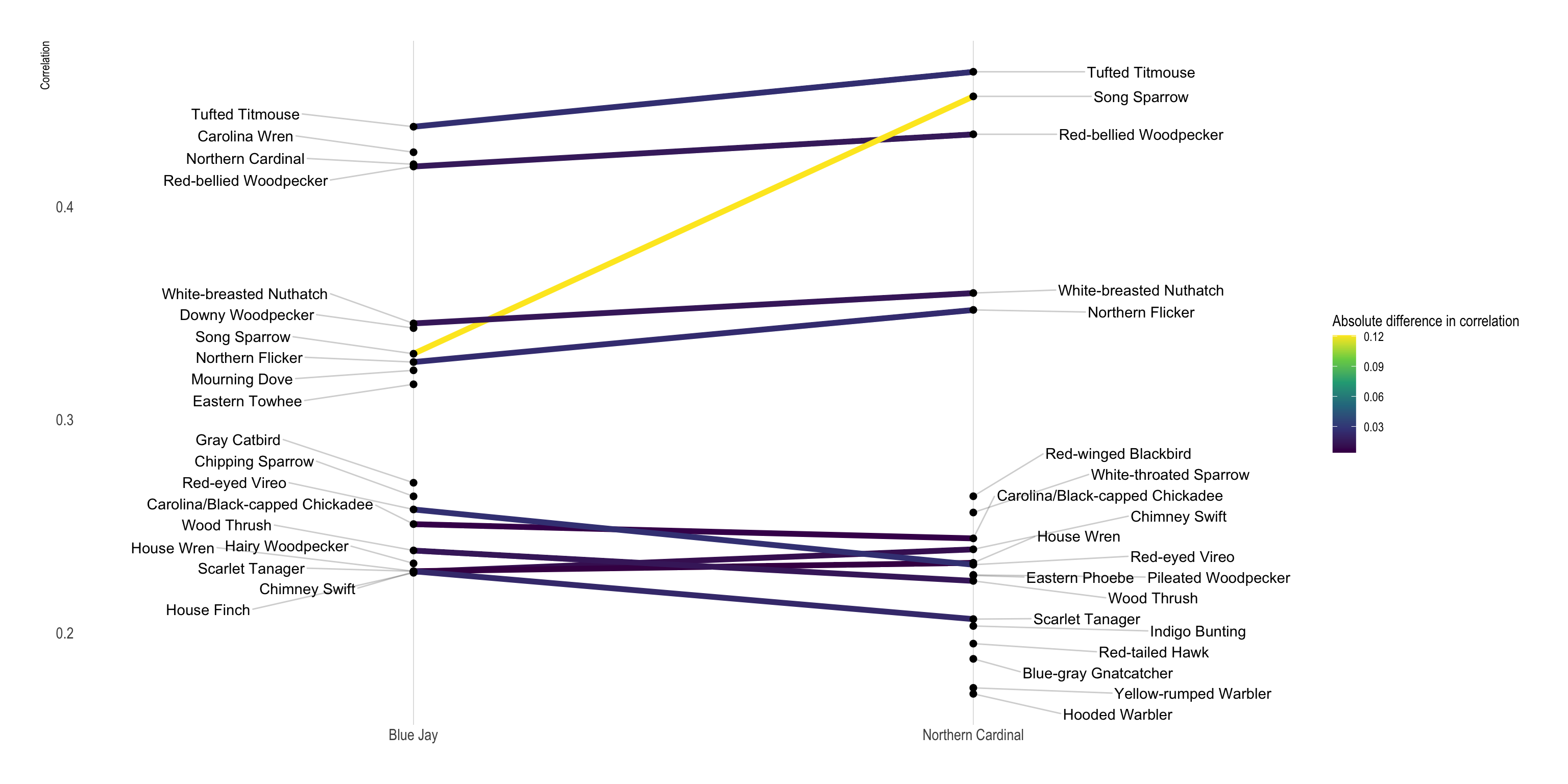## Network Graph

Network graphs are fun ways to show counts or correlations between groups. Since birds often exist in distinct environments and vary by season, graph analysis should be able to visualize connections.

This takes the top 100 birds in terms of total count and makes a network graph object consisting of nodes and edges.

``````graph_object_corr <- df_pair_corr %>%
semi_join(df_counts %>% slice(1:75), by = c("item1" = "common_name")) %>%
semi_join(df_counts %>% slice(1:75), by = c("item2" = "common_name")) %>%
as_tbl_graph(directed = FALSE) %>%
activate(nodes) %>%
filter(!node_is_isolated()) %>%
activate(edges) %>%
filter(abs(correlation) >= .05) %>%
activate(nodes) %>%
filter(!node_is_isolated()) %>%
left_join(df_counts, by = c("name" = "common_name"))

graph_object_corr``````
``````## # A tbl_graph: 74 nodes and 1921 edges
## #
## # An undirected simple graph with 1 component
## #
## # Node Data: 74 x 2 (active)
##   name                   species_count
##   <chr>                          <dbl>
## 1 American Crow                 143783
## 2 American Goldfinch             41307
## 3 American Robin                114062
## 4 Belted Kingfisher               2035
## 5 Black-capped Chickadee          9520
## 6 Blue Jay                       49700
## # … with 68 more rows
## #
## # Edge Data: 1,921 x 3
##    from    to correlation
##   <int> <int>       <dbl>
## 1     1     2       0.279
## 2     1     3       0.287
## 3     2     3       0.406
## # … with 1,918 more rows``````

This plots the network graph to show all the connections that fit the criteria in the code above:

``````plot <- graph_object_corr %>%
ggraph() +
geom_edge_link(aes(width = correlation, alpha = correlation)) +
geom_node_point(aes(size = species_count, alpha = species_count)) +
scale_size_continuous("Total observations", labels = scales::comma) +
scale_alpha_continuous("Total observations", labels = scales::comma) +
scale_edge_alpha("Observations together", range = c(.01, .3)) +
scale_edge_width("Observations together", range = c(.1, 2)) +
theme_void()

plot``````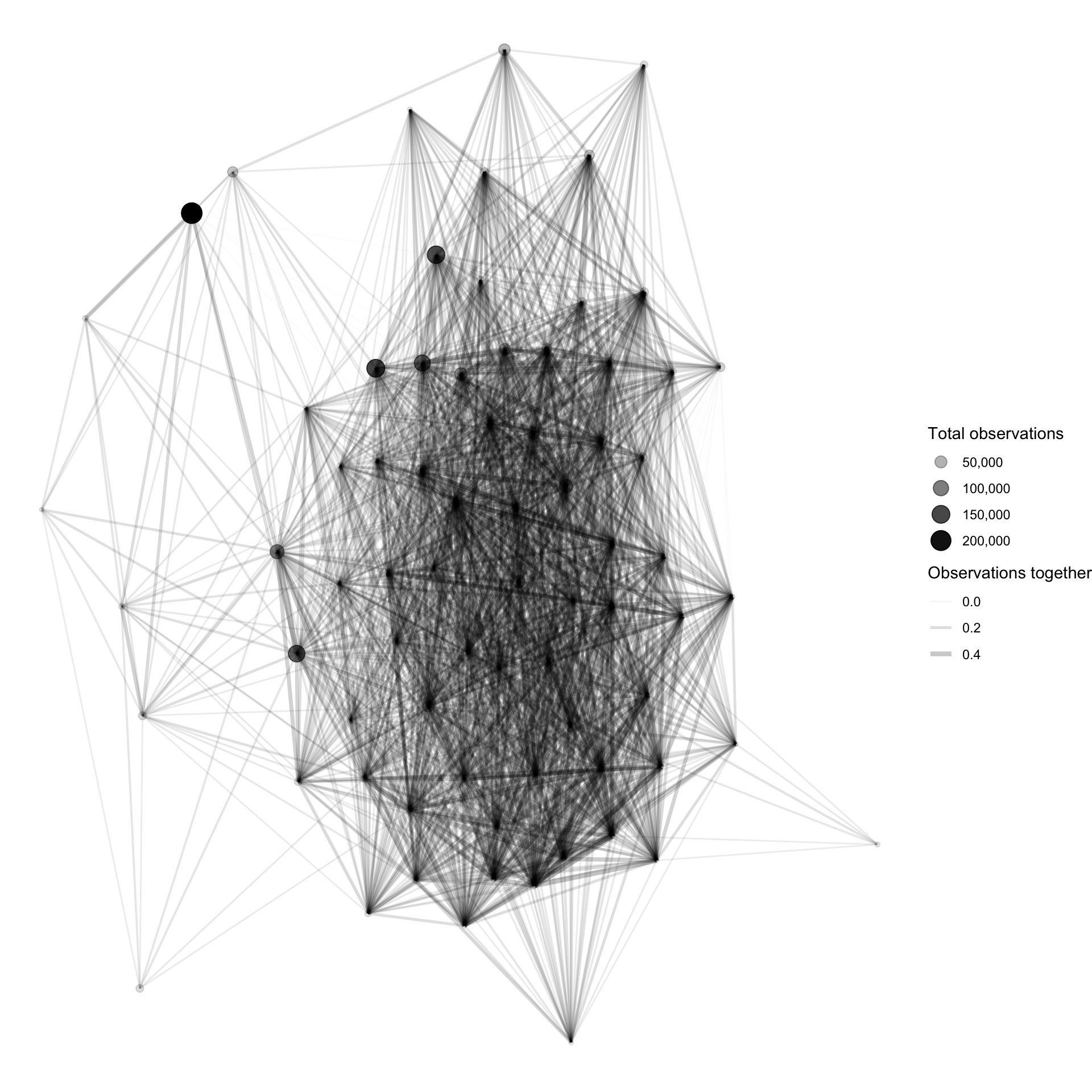There is a dense group of birds that are highly correlated with each other. This high correlation could be caused by a shared environment or seasonal migration patterns.

### Highlight species

I also wanted to be able to see where a given species fits in the network graph. This code prepares a network graph object and filters out species that are not highly connected to the main group.

``````graph_object_corr <- df_pair_corr %>%
as_tbl_graph(directed = FALSE) %>%
activate(edges) %>%
filter(abs(correlation) > .2) %>%
activate(nodes) %>%
mutate(centrality = centrality_authority()) %>%
filter(centrality > .01) %>%
filter(!node_is_isolated())``````

This code identifies the node ID for the Northern Cardinal and makes a dataframe I will use to filter with in the next code chunk.

``````species_list <- c("Northern Cardinal")

df_species_corr_nodes <- graph_object_corr %>%
activate(nodes) %>%
as_tibble() %>%
mutate(node_id = row_number()) %>%
filter(name %in% species_list)``````

This code identifes which node is the Northern Cardinal, and which edges connect to the Northern Cardinal. This identifies all the species that are connected to the bird, given the criteria I set above.

``````plot_corr <- graph_object_corr %>%
activate(nodes) %>%
mutate(name_label = case_when(name %in% species_list ~ name,
TRUE ~ as.character(NA))) %>%
activate(edges) %>%
left_join(df_species_corr_nodes, by = c("from" = "node_id")) %>%
left_join(df_species_corr_nodes, by = c("to" = "node_id")) %>%
mutate(name = coalesce(name.x, name.y),
centrality = coalesce(name.x, name.y)) %>%
select(-matches(".x|.y")) %>%
mutate(species_flag = case_when(from %in% df_species_corr_nodes\$node_id | to %in% df_species_corr_nodes\$node_id ~ TRUE,
TRUE ~ FALSE),
name = case_when(is.na(name) ~ "Other species",
TRUE ~ name)) %>%
ggraph() +
geom_edge_link(aes(alpha = species_flag, width = species_flag)) +
geom_node_point(aes(shape = !is.na(name_label), size = !is.na(name_label), color = name_label)) +
geom_node_label(aes(label = name_label, color = name_label), repel =  TRUE) +
scale_edge_width_manual(values = c(.3, 1)) +
scale_edge_alpha_discrete(range = c(0.1, .5)) +
scale_shape_manual(values = c(1, 19)) +
scale_size_manual(values = c(2, 3)) +
guides(edge_alpha = FALSE,
edge_width = FALSE,
size = FALSE,
shape = FALSE,
color = FALSE) +
theme_void()

plot_corr``````